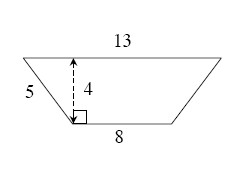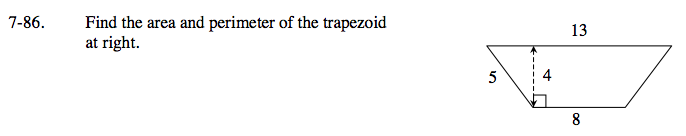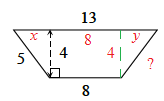### Home > GC > Chapter 7 > Lesson 7.2.5 > Problem7-86

7-86.
1. Find the area and perimeter of the trapezoid below. Homework Help ✎

2.Don't assume the trapezoid is isosceles even though it looks like it.
Start by solving for x in the diagram, then solve for y, then find the length of the unknown side of the trapezoid.Area = 42 square units